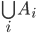Next Topology of Data Types 10

# Parallel computations

• Let's suppose our computation model allows parallel computations

• (Countably) infinite unions of semidecidable sets are semidecidable

• To semidecide:

• In parallel, try to semidecide each of the Ai

• If any is true, one of the semidecisions will succeed in finite time

• When it does, halt and say "YES"

• (Warning: There are some important qualifications here that I will skip over.)

• (Computer scientists must be much less cavalier than mathematicians about infinite operations.)

• (e.g., what if i ranges over a noncomputable subset of ℕ?)